## Introduction

The orbital angular momentum (OAM) of light has attracted considerable attention in recent years. As identified by Allen et al.1 in 1992, a beam of light with an azimuthal phase dependence of the formcarries OAM, whereis the mode index, which specifies the amount of OAM, and Φ is the azimuthal angle. This interesting property of light has been explored in different contexts. For example, fundamental tests of high-dimensional entangled systems have been performed through the OAM basis2, the infinite OAM bases have been used to implement paradoxes in quantum mechanics3 and relativistic effects have been explored in the azimuthal degree of freedom4, 5. In the applied context, the OAM of light has been used to encode information6, 7, 8, 9, 10, manipulate microscopic particles11, 12, 13, 14, perform optical metrology15, 16, and perform remote sensing and imaging4, 17, 18, 19, 20, 21, 22, 23, 24, 25.

It has been suggested that the discrete OAM spectrum (or spiral spectrum) can be used for imaging, a technique known as digital spiral imaging17. In addition, quantum OAM correlations26 have been used to enhance the image contrast of phase objects18. Furthermore, object identification has been performed by means of quantum-correlated OAM states19, 20. Similarly, quantum correlations have been incorporated into digital spiral imaging to retrieve information of phase objects21. Moreover, field correlations in vectorial beams have been utilized for kinematic sensing22.

It has been recently demonstrated that random fluctuations of light give rise to the formation of intensity correlations in the OAM components and angular positions of pseudothermal light27. It has also been shown that these classical correlations are manifested through interference structures that resemble those observed with entangled photons. These results suggest that OAM intensity correlations in random optical fields, such as those found in natural light, could be used to develop optical technologies with the similar functionality as those that employ entangled photons.

In this work, we exploit the OAM correlations of random light to demonstrate object identification; in this approach, the object is identified through its discrete OAM spectrum (or spiral spectrum). We also demonstrate that we can use the same types of correlations to retrieve the phase information of an object. Despite the fact that intensity correlations in the OAM degree of freedom are not perfect, as for the case of entangled photons, it is possible to perform object identification at any light levels, an important advantage over the quantum protocols that employ fragile entangled states of light.

## Materials and methods

### Theoretical analysis

The OAM spectrum of a random light field E(r,Φ) can be experimentally measured by projecting it onto a series of OAM modes. The amplitude for this projection is given byThe angular coherence properties of a field of light are described by the first-order correlation function, where the symbolindicates the ensemble average. Similarly, the second-order correlation function that describes intensity correlations in the OAM domain is defined as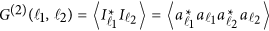. For a thermal beam of light,is given by27, 28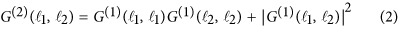The first term in Equation (2) represents a product of intensities between two OAM modes. This first term is constant and represents a background that causes the intensity correlations not to be perfect, whereas the second term,, is typically approximated by a discrete δ function that describes point-to-point OAM correlations.

In our scheme for object identification, one of the two beams illuminates the object described by the transmission function A(r,Φ). In this case, the second term of Equation (2), which is defined as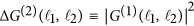, can be expressed (see the Supplementary Information) aswhere, and the overbar means ensemble average. Interestingly, the object A(r,Φ) encodes its Fourier components into the second-order correlation function. This signature is used to recover its spatial or phase information. When the object is not present, A(r,Φ)=1, and this term takes the form of a discrete δ function.

### Experimental setup

Figure 1a shows the experimental setup we use for digital spiral object identification. A 532-nm diode laser illuminates a digital micro-mirror device (DMD), which is used to generate a random field of light29, 30. A 4f-optical system consisting of two lenses and a spatial filter is employed to isolate the first order of the beam diffracted by the DMD. The intensity distribution of the generated beam is shown in Figure 1b.

The random light field is divided into ‘test’ and ‘reference’ arms after passing through a beam splitter. The light beam in the test arm interacts with an amplitude or phase object, which is displayed onto a spatial light modulator (SLM), as shown in Figure 1c. Each light beam is then projected onto a forked hologram to measure an OAM component of the random field of light19, 20, 21, 31. The first diffraction order of the structured beam is filtered by an aperture and then is coupled into a single mode fiber and detected by an avalanche photodiode (APD). Two APDs and a coincidence count module are utilized to measure OAM correlations between the two arms. The total accumulation time of each measurement is set to 5 s in our experiment.

## Results and discussion

### Amplitude object identification

As shown in Figure 2a and 2b, we use objects with four- and sixfold rotational symmetries. Each object is encoded onto the SLM located in the test arm.

A series of OAM projections is performed in each arm to construct a two-dimensional matrix with the normalized second-order correlation function (Figure 2c and 2d). The OAM number in the test and reference arms are denoted byand, respectively. The normalized second-order OAM correlation function is calculated by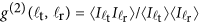, whereis proportional to the coincidence count rate. Each element in the matrix is obtained by averaging over 50 realizations of the experiment, and the error bars are obtained by calculating the standard deviation.

As shown in Figure 2c and 2d, an amplitude object with N-fold rotational symmetry imprints its Fourier components into the second-order OAM correlation matrix. The correlation signal is high along the diagonal elements of the matrix, where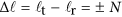due to the symmetry of the amplitude object. In our case, these signatures can be observed when, for the object with fourfold rotational symmetry, and when, for the object with sixfold rotational symmetry. Consequently, it is evident that one can use the OAM correlation matrix to identify the two objects. Furthermore, note that this technique requires a small number of measurements compared to traditional imaging schemes that rely on pixel-by-pixel raster scanning.

In Figure 2e and 2f, we plot the transverse sections, defined by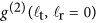, for the correlation matrices in Figure 2c and 2d, respectively. For simple and symmetric objects, a single line in the correlation matrix can provide adequate information about the object. However, the measurement of the total OAM correlation matrix is required for complicated objects that lack rotational symmetry19, 32.

### Phase object identification

We showed above that our technique is capable of identifying amplitude objects with rotational symmetry. Next, we demonstrate that our technique can also be used to identify phase objects. As a specific example, we use phase objects consisting of non-integer vortices described as eiMΦ, where M indicates a non-integer winding number33, 34, 35, 36, 37. The phase profile of a vortex with M=−2/3 is shown in Figure 3a; the azimuthal phase for a non-integer vortex of this form ranges from −2π/3 to 2π/3. The forked hologram that we encode onto the SLM is shown in Figure 3b. The two-dimensional normalized second-order OAM correlation matrix is shown in Figure 3c, and its middle row is plotted in Figure 3d. In this case, the presence of the phase object induces a broader spectrum in the correlation matrix.

As shown in Figure 4, we also test the performance of our technique with different phase objects characterized by the non-integer winding numbers M=−1/2, M=−5/2, M=−2/3 and M=−8/3. The performance of our technique can be characterized through the Floor function. This simple function is used to denote the largest previous integer of M and can be defined as, and v is the non-integer part given by v=M−u. The theoretical and experimental results show that the central peak of the correlation signal is determined by u and the profile is determined by v. A simple comparison between Figure 4a and 4b shows that the two figures have the same profile, but the central peak is located at different positions. This difference is because the parameter v is equal to 1/2 for both cases, whereas the parameter u is different; this parameter is equal to −1 and −3 for Figure 4a and 4b, respectively. We can compare the results shown in Figure 4c and 4d; in this case, the parameter v is equal to 1/3, whereas the parameter u is equal to −1 and −3 for Figure 4c and 4d, respectively. In this case, the two figures have the same profile, but the peak is located at different positions.